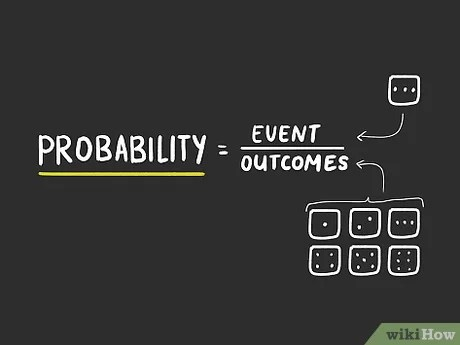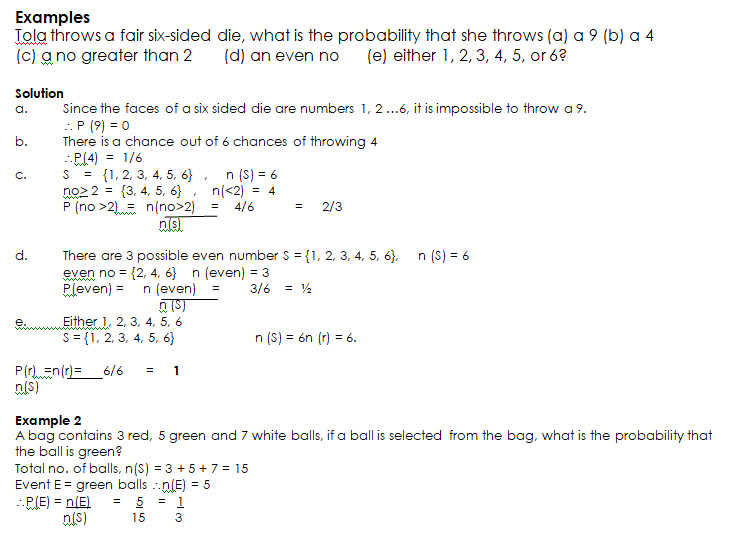# Probability

Welcome to class!

In today’s class, we will be talking about probability. Enjoy the class!

### Probability#### DEFINITION OF TERMS

(i)Event: When an experiment is performed two or more results or outcomes will be expected to happen. Each attempt is called a trial and the outcome of a trial and the outcome of a trial is called an event, usually denoted by E.

(ii)Random Experiment: A random experiment is a repetitive process which may result in any one of the possible outcomes of the experiment OR:

(iii)Sample space: The sample space of a random experiment is the set containing all the possible outcomes of the experiment OR:

Sample space is all the possible outcomes of a trial in an experiment usually denoted by S.

(iv)The number of the points in a sample space n(s), and in an event, E is n(E).EVALUATION

Use the figure below to answer the following:

 16 2 3 13 5 11 10 8 9 7 6 12 4 14 15 1

(a)      If a number is picked at random from the figure. What is the probability that it is:-

(i)        Odd          (ii) Prime     (iii)   even       (iv) less than 10

(v)       Exactly divisible by 3       (vi)        a perfect square   (vii)      a perfect cube?

(b)      If a row or column is picked at random from the figure. What is the probability that the total of its no is(i)  34         (ii)      35

###### GENERAL EVALUATION
1.  A bag contains black balls, 3 green balls and 4 red balls, A ball is picked form the bag at random, what is the probability that it is (a) Black       (d) yellow     (c) Green     (d) not black (d) either black ore red
2. A school contains 357 boys and 323 girls if a student is chosen at random, what is the probability that a girl is chosen.

In our next class, we will be talking more about probability.  We hope you enjoyed the class.

Should you have any further question, feel free to ask in the comment section below and trust us to respond as soon as possible.

How Can We Make ClassNotesNG Better - CLICK to Tell Us💃